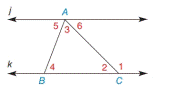Chapter 2.4, Problem 15EElementary Geometry For College St...

7th Edition
Alexander + 2 others
ISBN: 9781337614085

Solutions

Chapter
SectionElementary Geometry For College St...

7th Edition
Alexander + 2 others
ISBN: 9781337614085
Textbook Problem

In Exercises 13 to 15, j ∥ k and. Δ A B C . Given: m ∠ 1 = 122.3 ° m ∠ 5 = 41.5 ° Find: m ∠ 2 , m ∠ 3 , and m ∠ 4To determine

To find:

The measure of angles m2, m3, and m4.

Explanation

Given:

In ΔABC,

m1=122.3°m5=41.5°jk

Figure (1)

Properties used:

(1) In a triangle, the sum of the measures of all interior angles is 180°.

(2) If two parallel lines are cut by a transversal, then pairs of alternate interior angles are congruent.

(3) Angles on a straight line add up to 180°.

Calculation:

In ΔABC,

m1=122.3°m5=41.5°jk

4 and 5 are two alternate interior angles.

So,

45

Thus,

m4=m5

Substitute 41.5° for m5 in the above equation.

m4=41.5°

1 and 2 are angles on a straight line

Still sussing out bartleby?

Check out a sample textbook solution.

See a sample solution

The Solution to Your Study Problems

Bartleby provides explanations to thousands of textbook problems written by our experts, many with advanced degrees!

Get Started

In Exercises 1-6, solve for y. y=15x

Calculus: An Applied Approach (MindTap Course List)

Solve the equations in Exercises 126. 14x2=0

Finite Mathematics and Applied Calculus (MindTap Course List)

The third partial sum of is:

Study Guide for Stewart's Single Variable Calculus: Early Transcendentals, 8th